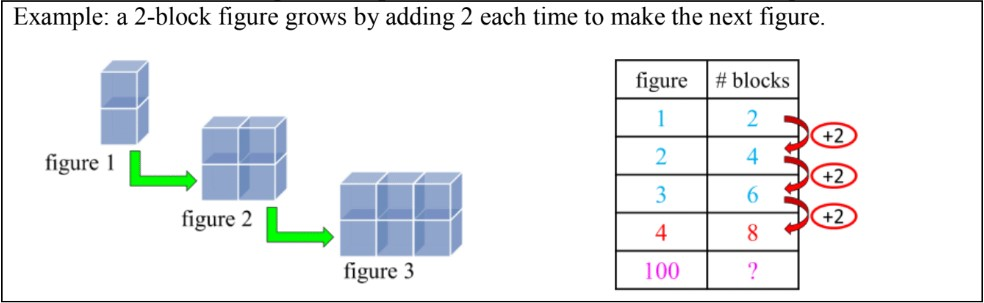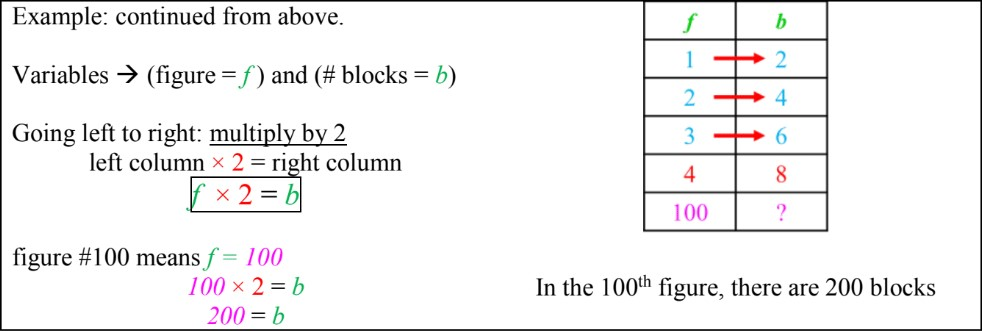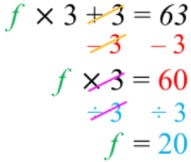# Introduction to solving patterns in T-tables and equations#### Everything You Need in One Place

Homework problems? Exam preparation? Trying to grasp a concept or just brushing up the basics? Our extensive help & practice library have got you covered.#### Learn and Practice With Ease

Our proven video lessons ease you through problems quickly, and you get tonnes of friendly practice on questions that trip students up on tests and finals.#### Instant and Unlimited Help

Our personalized learning platform enables you to instantly find the exact walkthrough to your specific type of question. Activate unlimited help now!

0/4
##### Intros
###### Lessons
1. Introduction to Solving Patterns in T-tables and Equations:
2. Finding one-step relationships between columns in a T-table
3. Writing equations for T-table relationships (one-step)
4. Writing equations for T-table relationships (two-step)
5. How to isolate variables in two-step equations (solving algebra equations)
0/16
##### Examples
###### Lessons
1. T-tables and one-step equations
Write the equation for the relationship between the two variables. Follow the pattern to complete the T-table.
1. variables $a$ and $b$
 a b 1 14 2 15 3 16 4 20
2. variables $f$ and $g$
 f g 50 28 49 27 48 26 47 25
3. variables $q$ and $r$
 q r 8 32 9 36 10 40 11 1
4. variables $v$ and $w$
 v w 27 9 24 8 21 7 18 3
2. T-tables and two-step equations
Write the equation for the relationship between the two variables. Follow the pattern to complete the T-table.
1. variables $i$ and $j$
 i j 1 3 2 5 3 7 4 10
2. variables $d$ and $e$
 d e 5 13 6 16 7 19 8 1
3. variables $x$ and $y$
 x y 20 13 18 12 16 11 14 100
4. variables $x$ and $y$
 x y 24 4 21 3 18 2 15 300
3. T-tables and equations: word problem - 1
It costs $8 to rent a bicycle for the first hour. It costs$2 every hour after that.
1. Fill out a T-table for the cost of the first five hours.
 hour cost
2. Write an equation with variables for the relationship between hours ($h$) and the cost ($C$).
3. How much would it cost to rent a bicycle for 24 hours?
4. If someone paid a total of \$26, how many hours did they rent a bicycle for?
4. T-tables and equations: word problem - 2
After the first month, seed A grew into a 2cm plant and continued to grow 5cm each month after that. Seed B grew to 6cm and continued to grow 3 cm each month after that.
1. Fill out a T-table for the cost of the first three months for both seeds.
 month heightA
 month heightB
2. Write an equation for each seed's growth, relating the number of months ($m$A, $m$B) and the height ($H$A, $H$B).
3. After 1 year, what is the height difference between the two plants?
4. When will each plant reach 42cm in height?
0%
##### Practice
###### Free to Join!
StudyPug is a learning help platform covering math and science from grade 4 all the way to second year university. Our video tutorials, unlimited practice problems, and step-by-step explanations provide you or your child with all the help you need to master concepts. On top of that, it's fun - with achievements, customizable avatars, and awards to keep you motivated.
• #### Easily See Your ProgressWe track the progress you've made on a topic so you know what you've done. From the course view you can easily see what topics have what and the progress you've made on them. Fill the rings to completely master that section or mouse over the icon to see more details.
• #### Make Use of Our Learning Aids###### Practice Accuracy

See how well your practice sessions are going over time.

Stay on track with our daily recommendations.

• #### Earn Achievements as You LearnMake the most of your time as you use StudyPug to help you achieve your goals. Earn fun little badges the more you watch, practice, and use our service.
• #### Create and Customize Your AvatarPlay with our fun little avatar builder to create and customize your own avatar on StudyPug. Choose your face, eye colour, hair colour and style, and background. Unlock more options the more you use StudyPug.
###### Topic Notes

In this lesson, we will learn:

• How to determine and write one-step and two-step relationships in T-tables as equations with variables
• How to solve equations: isolating variables in two-step relationships (opposite operation to both sides)

Notes:

• Recall that T-tables keep track of patterns and allow calculation of subsequent terms• What is a fast way to find the 100th term? Adding by 2 each time is not efficient.
• T-table columns have a relationship between them. By writing this relationship as an equation, you can put in any number and find information about that term quickly.
• Ask: how do you go from the left column to the right column?
• Find one-step relationships through trial and error (if numbers are getting bigger it is + or ×; if numbers are getting smaller it is - or ÷)
• Assign variables to each column and use these variables in your equation• For more complicated patterns (two-step relationships) the equation will have two operations: the first step will be [× or ÷] and then the second step will be [+ or -]
• Similar to one-step relationships, they can be found through trial and error

• Isolating variables in two-step equations by “doing the opposite to both sides” in the backwards order of operations (solve by doing + or - first and then × or ÷ after)